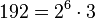# Groups of order 192

Jump to: navigation, search

## Contents

This article gives information about, and links to more details on, groups of order 192
See pages on algebraic structures of order 192| See pages on groups of a particular order

## Statistics at a glance

To understand these in a broader context, see groups of order 3.2^n

The number 192 has prime factorization$192 = 2^6 \cdot 3$. There are only two prime factors of this number. Order has only two prime factors implies solvable (by Burnside's$p^aq^b$-theorem) and hence all groups of this order are solvable groups (specifically, finite solvable groups). Another way of putting this is that the order is a solvability-forcing number. In particular, there is no simple non-abelian group of this order.

Quantity Value Explanation
Total number of groups 1543
Number of abelian groups 11 (number of abelian groups of order$2^6$) times (number of abelian groups of order$3^1$) = (number of unordered integer partitions of 6) times (number of unordered integer partitions of 1) =$11 \times 1 = 11$. See classification of finite abelian groups and structure theorem for finitely generated abelian groups.
Number of nilpotent groups 267 (number of groups of order 64) times (number of groups of order 3) =$267 \times 1 = 267$. See number of nilpotent groups equals product of number of groups of order each maximal prime power divisor, which in turn follows from equivalence of definitions of finite nilpotent group.
Number of solvable groups 1543 There are only two prime factors of this number. Order has only two prime factors implies solvable (by Burnside's$p^aq^b$-theorem) and hence all groups of this order are solvable groups (specifically, finite solvable groups). Another way of putting this is that the order is a solvability-forcing number. In particular, there is no simple non-abelian group of this order.
Number of simple groups 0 Follows from all groups of this order being solvable.

## GAP implementation

The order 192 is part of GAP's SmallGroup library. Hence, any group of order 192 can be constructed using the SmallGroup function by specifying its group ID. Also, IdGroup is available, so the group ID of any group of this order can be queried.

Further, the collection of all groups of order 192 can be accessed as a list using GAP's AllSmallGroups function.

Here is GAP's summary information about how it stores groups of this order, accessed using GAP's SmallGroupsInformation function:

gap> SmallGroupsInformation(192);

There are 1543 groups of order 192.
They are sorted by their Frattini factors.
1 has Frattini factor [ 6, 1 ].
2 has Frattini factor [ 6, 2 ].
3 - 4 have Frattini factor [ 12, 3 ].
5 - 126 have Frattini factor [ 12, 4 ].
127 - 179 have Frattini factor [ 12, 5 ].
180 - 187 have Frattini factor [ 24, 12 ].
188 - 204 have Frattini factor [ 24, 13 ].
205 - 806 have Frattini factor [ 24, 14 ].
807 - 943 have Frattini factor [ 24, 15 ].
944 - 991 have Frattini factor [ 48, 48 ].
992 - 1019 have Frattini factor [ 48, 49 ].
1020 - 1025 have Frattini factor [ 48, 50 ].
1026 - 1399 have Frattini factor [ 48, 51 ].
1400 - 1467 have Frattini factor [ 48, 52 ].
1468 - 1488 have Frattini factor [ 96, 226 ].
1489 - 1495 have Frattini factor [ 96, 227 ].
1496 - 1504 have Frattini factor [ 96, 228 ].
1505 - 1509 have Frattini factor [ 96, 229 ].
1510 - 1529 have Frattini factor [ 96, 230 ].
1530 - 1536 have Frattini factor [ 96, 231 ].
1537 - 1543 have trivial Frattini subgroup.

For the selection functions the values of the following attributes
are precomputed and stored:
IsAbelian, IsNilpotentGroup, IsSupersolvableGroup, IsSolvableGroup,
LGLength, FrattinifactorSize and FrattinifactorId.

This size belongs to layer 2 of the SmallGroups library.
IdSmallGroup is available for this size.